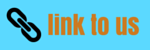# Change of State of Matter

Notes NCERT Solutions

## The Common Unit of Temperature and SI Unit of Temperature:

• The common unit of measuring temperatures (like melting points, boiling points etc.) is ‘degrees Celsius’ which is written in short form as °C.
• Laboratory thermometers and clinical thermometer are calibrated on Celsius scale of temperature.
• There is another scale of temperature called Kelvin scale of temperature which is used by the scientists mainly for research work. The SI unit of measuring temperature is Kelvin, which is denoted by the symbol K.
• The relation between Kelvin scale and Celsius scale of temperature can be written as:
Temp. on Kelvin scale=Temp. on Celsius scale + 273
1. To convert a temperature on Celsius scale to Kelvin scale, we have to add 273 to the Celsius temperature.
2. And to convert a temperature on Kelvin scale to the Celsius scale, we have to subtract 273 from the Kelvin temperature.

## Change of State of Matter:

• We can change the physical state of matter in two ways:
1. By changing the temperature (heating or cooling).
2. By changing the pressure (increasing or decreasing the pressure).
• These two factors decide whether a given substance would be in a solid, liquid or gaseous state.

## Effect of Change of Temperature:

### Solid to Liquid Change: Melting

• The process in which a solid substance changes into a liquid on heating,is called on melting or fusion.
• The temperature at which a solid substance melts and changes into a liquid at atmospheric  pressure,is called melting point of substance. For example, the ice melts at a temperature of 0°C to form liquid water, so the melting point of ice is 0°C (zero degree Celsius). At melting point, ice changes its state from solid to liquid.
• Different solids have different melting points. Higher the melting point of a solid substance, greater will be the force of attraction between its particles.
• The melting point of a solid is an indication of the strength of the force of attraction between its particles.
• When a solid substance is heated, the heat energy makes its particles vibrate more vigorously. At the melting point, the particles of a solid have sufficient kinetic energy to overcome the strong forces of attraction holding them in fixed positions and break to form small groups of particles and the solid melts to form a liquid.

### Liquid to Gas Change: Boiling (or Vaporisation):

• The process in which a liquid substance changes into a gas rapidly on heating, is called boiling.
• The temperature, at which a liquid boils and changes rapidly into a gas at atmospheric pressure, is called boiling point of the liquid.
• For example, when water is heated to a temperature of 100 °C (hundred degree Celsius).
• Different liquids have different boiling points.
• The boiling point of a liquid is a measure of the force of attraction between its particles.
• When a liquid is heated, the heat energy makes its particles move even faster. At the boiling point the particles of liquid have sufficient kinetic energy to overcome the forces of attraction holding them together and separate into individual particles. And the liquid boils to form a gas.

### Gas to Liquid Change: Condensation

• The process of changing a gas (or vapour) to a liquid by cooling is called condensation.
• When steam (or water vapour) changes into water on cooling, it is called condensation of steam.
• When a gas is cooled enough, then its particles lose so much kinetic energy that they slow down, move closer together until they start being attracted to each other, and form a liquid.

### Liquid to solid change: Freezing

• The process of changing a liquid into a solid by cooling is called freezing.
• For example, when water is cooled, it gets converted into a solid called ‘ice’. This is called freezing of water. Freezing means solidification.